Mastering the Euler Phi Function:

Exercise 1.

a)      Compute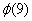and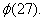b)      Use a counting argument to prove that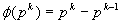for p prime and k a positive integer.

Exercise 2.

Our goal now is to prove the following theorem:

# Theorem

If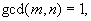then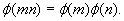a)      Recalling that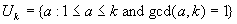and assuming that, prove that the mapping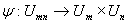defined by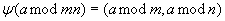is one-to-one.

b)      Assuming that the “Baby Chinese Remainder Theorem” stated below is true, prove that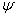is onto.

# The “Baby” Chinese Remainder Theorem

Let m and n be integers with gcd(m, n) =1, and let b and c be any integers.  Then the simultaneous congruences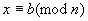and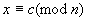have exactly one solution with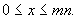c)      Use parts a) and b) to explain why you now know that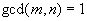impliesExercise 3.  Compute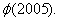Functions satisfying this criteria (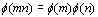whenever gcd(m, n) = 1) are said to be multiplicative.

 Don’t worry…we will prove this theorem and tackle the “Adult” version next lesson!

Back to Block 1 Course Assignments